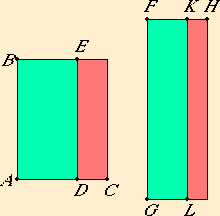# Proposition 109

If a rational area is subtracted from a medial area, then there arise two other irrational straight lines, either a first apotome of a medial straight line or a straight line which produces with a rational area a medial whole.

Let the rational area BD be subtracted from the medial area BC.

I say that the side of the remainder EC becomes one of two irrational straight lines, either a first apotome of a medial straight line or a straight line which produces with a rational area a medial whole.X.13

Set out a rational straight line FG, and apply the areas similarly. Then FH is rational and incommensurable in length with FG, while KF is rational and commensurable in length with FG, therefore FH and FK are rational straight lines commensurable in square only.

X.73

Therefore KH is an apotome, and FK the annex to it.

Now the square on HF is greater than the square on FK either by the square on a straight line commensurable with HF or by the square on a straight line incommensurable with it.

X.Def.III.2

If the square on HF is greater than the square on FK by the square on a straight line commensurable with HF, while the annex FK is commensurable in length with the rational straight line FG set out, then KH is a second apotome.

X.92

But FG is rational, so that the side of LH, that is, of EC, is a first apotome of a medial straight line.

But, if the square on HF is greater than the square on FK by the square on a straight line incommensurable with HF, while the annex FK is commensurable in length with the rational straight line FG set out, then KH is a fifth apotome, so that the side of EC is a straight line which produces with a rational area a medial whole.

Therefore, if a rational area is subtracted from a medial area, then there arise two other irrational straight lines, either a first apotome of a medial straight line or a straight line which produces with a rational area a medial whole.

Q.E.D.

## Guide

This proposition is not used in the rest of the Elements.